# How to calculate degree ?369

If tan(X) = 20.

What function use to find X ?

Thank you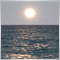5624310

## How to calculate degree?

Angles are are a measure of X/Y, same units, therefor a dimensionless value ,(which is what Tan(x) gives you.)
Angle on a chart is meaningless. We have price vs time. What is the angle of going 5 miles in 10 minutes? Meaningless! You can only calculate a slope on a chart.2397

WHRoeder:

## How to calculate degree?

Angles are are a measure of X/Y, same units, therefor a dimensionless value ,(which is what Tan(x) gives you.)
Angle on a chart is meaningless. We have price vs time. What is the angle of going 5 miles in 10 minutes? Meaningless! You can only calculate a slope on a chart.
Not true. You can calculate the angle of inclination of any line that can be represented by x,y co-ordinates regardless of the units of measurement.24310

 SDC: Not true. You can calculate the angle of inclination of any line that can be represented by x,y co-ordinates regardless of the units of measurement. No, you can not. ArcTan takes a dimensionless number. The units mater. Now answer the question "What is the angle of going 5 miles in 10 minutes?" Meaningless! Answer the question, what is the angle of climbing 10 stories in 5 minutes. Meaningless!No, you can not. Draw a line on your chart. Guestimate the angle. Now change the vertical scale. Is the angle the same? Absolutely not. Meaningless!2397

You are denying what is right in front of your face. If you cannot create a corrolation between two different units of measurement, how can you create a forex chart using time and price ? How can you write code to draw indicator lines on such meaningless values ?

When you calculate the angle you are not saying what is the angle of going 5 miles in 10 minutes, you are saying what is the angle of the line on the chart you have created, reletive to its x axis.

The math can be applied to any two sets of values that you wish to corrolate on a chart, regardless of their units of measurement. I did not invent the math it is established and proven.

Of course the angle changes if you mess with the scale. Calculate the angle from a point 10 feet down your yard to the apex of your house. Now change that to 10 meters down your yard. Is it impossible to calculate an angle from a point in your yard to the apex of your house, just because it is possible to change the scale ?  What happens when you change the scale on your forex chart from 1hr to 4hr, the whole chart is different, does that make it meaningless ?

From Wikipedia: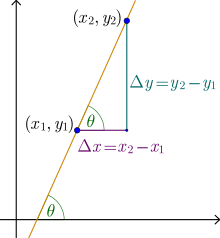The slope of a line in the plane containing the x and y axes is generally represented by the letter m, and is defined as the change in the y coordinate divided by the corresponding change in the x coordinate, between two distinct points on the line.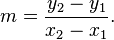The angle θ between -90° and 90° that a line makes with the x-axis is related to the slope m as follows: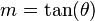and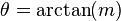(the inverse function of tangent).

For example, consider a line running through the points (2,8) and (3,20). This line has a slope, m, of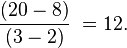The angle θ between -90° and 90° that this line makes with the x axis is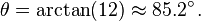17348

https://forum.mql4.com/59930/page3

You are still wrong . . .  and angle is specified in units of arc,  be it degree or radians . . . or anything else . . .  but it does not specify the units of the measurements of the 2 lines that make the angle.  So given an angle we have to assume that the units of the 2 lines is the same,  we have no information to do otherwise.2397

You think you proved me wrong because you asked a trick question on that other thread ? That is how the flat earth society make their case.

I guess I am going to have to write a script to prove this, I'll post it later.2397

OK Place a trendline on a chart. Name it "trend1" then run this script. You can move the line, zoom the chart etc then run it again. You will see I am not wrong.

```//+------------------------------------------------------------------+
//|                                                        Angle.mq4 |
//+------------------------------------------------------------------+
//| This script requires a trendline be already placed on the chart. |
//| the trendline should be named "trend1"                           |
//| Run the script and it will find the angle of that trendline      |
//| reletive to the x axis of the chart.                             |
//| To verify if the calculation is accurate, it will attempt to     |
//| place text at the same angle as the line.                        |
//| I used a trendline for convenience, the same code can be applied |
//| to any kind of a line with price time co-ordinates.              |
//+------------------------------------------------------------------+
#property version   "1.00"
#property strict
input string name = "trend1"; //Name of the Trendline
//+------------------------------------------------------------------+
//| Script program start function                                    |
//+------------------------------------------------------------------+
void OnStart()
{
//---
int x1=0,x2=0;
int y1=0,y2=0;
long chart = ChartGetInteger(0,CHART_HEIGHT_IN_PIXELS,0);
long t1=ObjectGetInteger(0,name,OBJPROP_TIME,0);
double p1=ObjectGetDouble(0,name,OBJPROP_PRICE,0);
long t2=ObjectGetInteger(0,name,OBJPROP_TIME,1);
double p2=ObjectGetDouble(0,name,OBJPROP_PRICE,1);
ChartTimePriceToXY(0,0,t1,p1,x1,y1);
ChartTimePriceToXY(0,0,t2,p2,x2,y2);
int size = (int)chart;
y1=size-y1;
y2=size-y2;
double angle =(MathArctan(((double)y2-(double)y1)/((double)x2-(double)x1))*180)/M_PI;
string textangle = DoubleToStr(angle,1);
string text = StringConcatenate("The Angle of This Line is ",textangle,"°");
ObjectCreate(0,"sometext",OBJ_TEXT,0,0,0);
ObjectSet("sometext",OBJPROP_TIME1,t1);
ObjectSet("sometext",OBJPROP_PRICE1,p1);
ObjectSetInteger(0,"sometext",OBJPROP_ANCHOR,ANCHOR_LEFT_LOWER);
ObjectSetString(0,"sometext",OBJPROP_TEXT,text);
ObjectSetDouble(0,"sometext",OBJPROP_ANGLE,angle);
ObjectSet("sometext",OBJPROP_COLOR,clrAqua);
ObjectSet("sometext",OBJPROP_FONTSIZE,18);
}
//+------------------------------------------------------------------+

```32002397

Yes because your trendline is running almost vertically downwards, the line's direction is defined by it's two parameters, the first parameter is the line's origin. As the angle is relative to the x axis, 90 degree is vertically up, -90 degrees is vertically down.